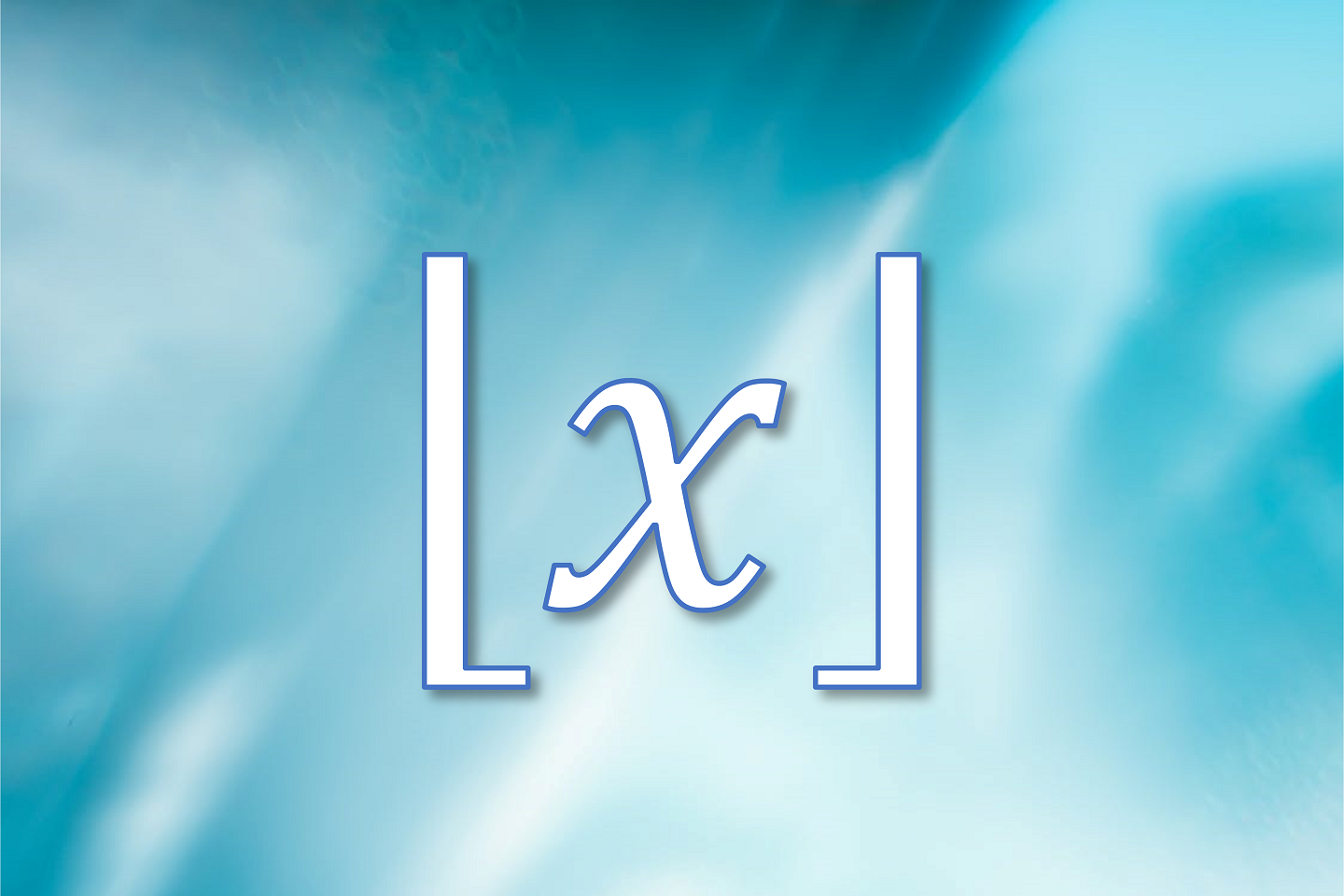# Throw Outdated Notation to the Floor

September 8, 2014
Mathematics
Teaching

There's no excuse for bad notation.

Well, there used to be.  In older times, mathematical notation and terminology were anything but standard - especially when the mathematics behind them were just coming into existence.  What Newton called fluxions, Leibniz called derivatives.  Even the now-ubiquitous $$\pi$$ was one of many symbols used to represent the constant $$3.14159...$$ by various mathematicians - and even then they weren't aware they only had half of the most fundamental circle constant. But as the human species has progressed, so has our ability to communicate with each other, allowing us to collaborate and spread ideas more quickly and more extensively than ever before.  It's no wonder that with the acceleration in communications technology, mathematicians have replaced clunky symbols with more elegant and consistent notations.

The only problem is that the math textbooks haven't quite caught up.

Let's take a look at a function that causes glazed-over eyes for many high schoolers every time it shows up in an Algebra II or Precalculus book:  the greatest integer function, usually notated as $$\left[x\right]$$ or occasionally $$\left[\!\left[x\right]\!\right]$$.  This function is defined as "the greatest integer that is less than or equal to $$x$$."  For example:

\begin{align*} \left[5\right]&=5\\ \left[5.1\right]&=5\\ \left[5.9\right]&=5\\ \left[-5.1\right]&=-6 \end{align*}
The bracket notation was invented by Carl Friedrich Gauss, who used it in his 1808 proof of the law of quadratic reciprocity.*

If you've taught high school students this function, you're probably familiar with the looks of confusion that come shortly after writing down the definition.  "So wait, do we want something less than the number or greater than the number?"  You could break down that definition piece by piece and hope your students can follow the roundabout logic, but perhaps you've found it's easier to link it to something that students are more familiar with - rounding.  To find $$\left[x\right]$$, all we need to do is round $$x$$ down to the nearest integer (meaning to the left on the real number line).  And of course, if $$x$$ is already an integer, then $$\left[x\right]=x$$, since no rounding needs to happen.

So now it's a bit easier to describe $$\left[x\right]$$, but the notation seems rather arbitrary, doesn't it?  We already use square brackets for other things - mainly for grouping terms together in large expressions and to denote closed intervals.  Writing $$\left[\!\left[x\right]\!\right]$$ is at least unique, but even more clunky.  And that's what Kenneth Iverson, the Canadian computer scientist who invented APL in 1962, must have thought as well.  In his book A Programming Language, he gave the function a new name - the floor function - and a new notation:

$\lfloor x\rfloor$
Now there's some solid notation!  The name and the bottom half-brackets suggest exactly what to do: round $$x$$ down to the nearest integer, just as described earlier.  Take a look:

\begin{align*} \lfloor5\rfloor&=5\\ \lfloor5.1\rfloor&=5\\ \lfloor5.9\rfloor&=5\\ \lfloor-5.1\rfloor&=-6 \end{align*}
I showed this to my own students, most of whom vaguely remembered the "greatest integer function" and only half of whom knew which direction it went.  It stuck.  The light bulbs went off all around the room, and the comments were to the effect of "well, that makes a lot more sense!" and "why didn't they teach it this way in the first place?"

But it gets better.  Mathematics is all about patterns and symmetry.  One of the students asked, "so if there's a floor function, is there a ceiling function?"  Yes there is.  The ceiling function is defined as "the least integer that is greater than or equal to $$x$$", and does exactly what you'd expect - it rounds the number up to the nearest integer.  Can you guess what the notation is?

$\lceil x\rceil$
And can you guess the answers to the following problems?

\begin{align*} \lceil5\rceil&=?\\ \lceil5.1\rceil&=?\\ \lceil5.9\rceil&=?\\ \lceil-5.1\rceil&=? \end{align*}
If you guessed $$5$$, $$6$$, $$6$$, and $$-5$$, you're correct.  See how easy math can be when the notation is evocative of the concept behind it?

At this point, you may be wondering, as you should:  why aren't textbooks aren't using this notation, given its obvious pedagogical advantage?  If this notation were only invented in the past few years or so, it might be excusable that the publishers haven't caught up yet.  But come on.  It's been over 50 years.  And with the way that textbook companies churn out new editions as often as they can, you'd think that one reason to do so would be to keep their math and their teaching up-to-date.

...right?

It seems that many people are under the unfortunate impression that math, unlike science or social studies, is already set in stone and nothing new really ever comes out of it.  This impression couldn't be further from the truth.  Mathematics is an ever-growing and ever-evolving body of knowledge.

And our potential to understand it better - and teach it better - hasn't hit the ceiling yet.

To read further about the floor and ceiling functions, visit the Wikipedia article on the subject.

* The law of quadratic reciprocity is a theorem in number theory.  Gauss thought it was so profound and beautiful that he occasionally referred to it as the "golden theorem".